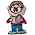## Tuesday, December 22, 2009

### Letter Equations 0 to 2

0 = L in T.

1 = K E.
1 = C A in M.
1 = W on a U.
1 = H on a U.
1 = R A in E B.
1 = D at a T.
1 = G L for M.

2 = H in a W.
2 = B of C in a M.
2 = S of R in K R B.
2 = number it T to T.
2 = R on a T.
2 = S to an A.
2 = F of J.
2 = Q in a C.

1.Can only get a few for now (and some probably wrong!), 0 Loss in Trying, 1 Wheel on a Unicycle, 1 Horn on a Unicorn, 1 Day at a Time, 2 Halves in a Whole, 2 Rails on a Track, 2 Sides to an Argument, 2 Faces of Janus, 2 Queens in a Chess (game)

2.0=L in T- Love in Tennis
1=K E ??
1=W on a U- Wheel on a Unicycle
1=H on a U- Horn on a Unicorn
1=R A in E B- Rotten Apple in Every Barrel
1=D at a T- Day at a Time
1=G L for M- Giant Leap for Mankind

2= H in a W ??
2= B of C in a M ??
2=S of R in K R B- Scoops of Rasins in Kellogs Raisin Bran
2=number it T to T- number it Takes to Tango
2= R on a T ??
2= S to an A ??
2= F of J ??
2= Q in a C ??

3.This comment has been removed by the author.

4.0 = L in T. Love in Tennis

1 = K E. Kelley's Eye (comic and bingo term)
1 = C A in M. Carbon Atom in Methane
1 = W on a U. Wheel on a Unicycle
1 = H on a U. Horn on a Unicorn
1 = R A in E B. Rotten Apple in Every Barrel
1 = D at a T. Day at a Time
1 = G L for M. Giant Leap for Mankind

2 = H in a W. 2 Halves in a Whole
2 = B of C in a M. Bottles of Champagne in a Magnum
2 = S of R in K R B. Scoops of Raisins in Kellog's Raisin Bran
2 = number it T to T. number it Takes to Tango
2 = R on a T. Rails on a Track
2 = S to an A. Sides to an Argument
2 = F of J. Faces of Janus
2 = Q in a C. Two quavers in a crotchet

Leave your answer or, if you want to post a question of your own, send me an e-mail. Look in the about section to find my e-mail address. If it's new, I'll post it soon.

Please don't leave spam or 'Awesome blog, come visit mine' messages. I'll delete them soon after.

Enter your Email and join hundreds of others who get their Question of the Day sent right to their mailbox

The Lamplight Manor Puzz 3-D